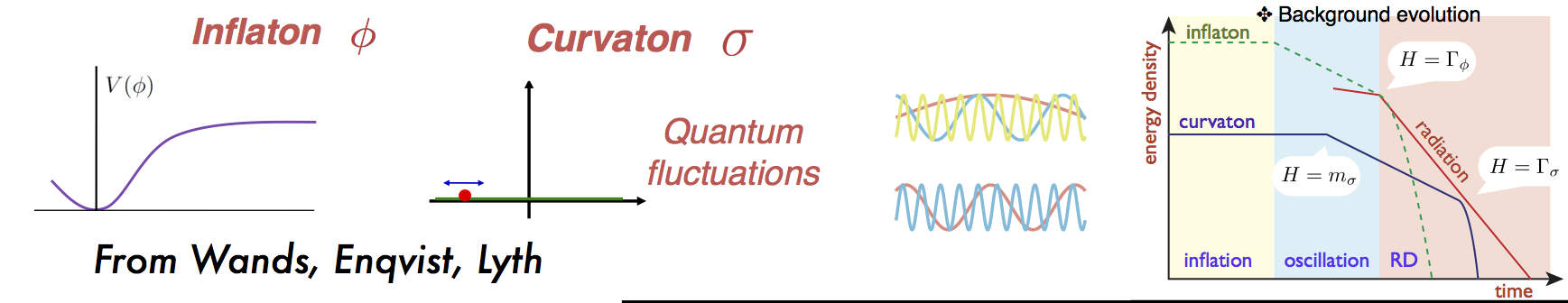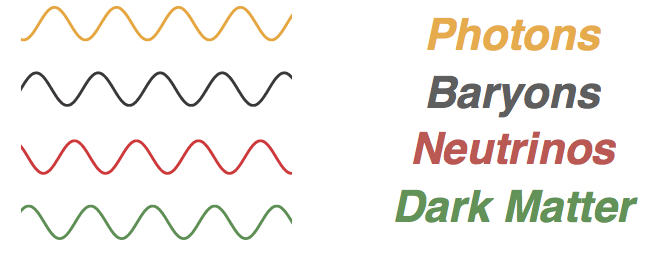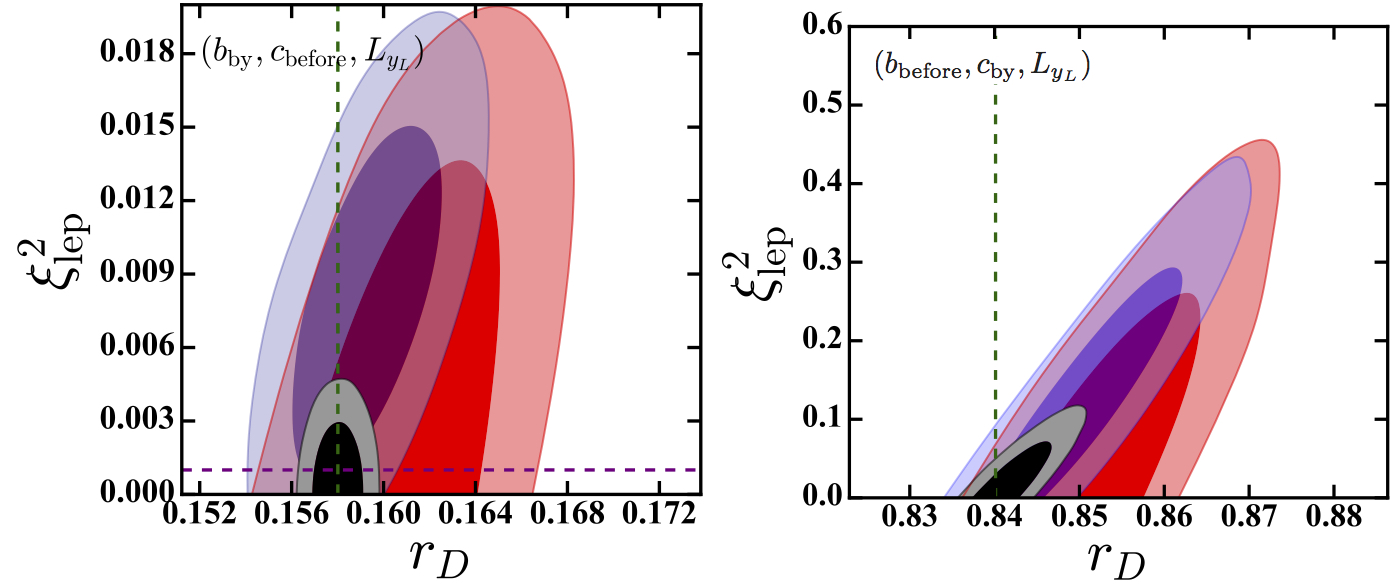Probing a panoply of curvaton-decay scenarios using CMB dataIn the curvaton model, an energetically sub-dominant field (the curvaton) during inflation eventually begins to redshift as matter, allowing it and its decay products to later dominate the overall primordial density fluctuation  (overtaking that caused by fluctuations in the inflaton itself). In this work, Tristan Smith and I systematically probed and limited the curvaton parameter space for all feasible curvaton-decay scenarios, using Planck data, an appropriately modified version of the CAMB code, and the parameter space estimation code CosmoMC. Depending on precisely how the curvaton decays into other particles, primordial fluctuations are an admixture of adiabatic (total density) and entropy (isocurvature fluctuations), meaning that fluctuations of the same wavelength in different species are not spatially in phase. This allows extremely precise Planck
satellite measurements of the CMB temperature/polarization power spectra to sensitively probe the curvaton parameter space, described by$r_{\rm D}$, and the lepton asymmetry of the universe, parameterized by the lepton chemical potential$\xi_{\rm lep}$, which sets the radiative energy density of the universe at the CMB surface of last scattering and the amplitude of neutrino isocurvature perturbations.

Result on the arXiv (under review by Phys. Rev. D): arXiv:1511.07431.

In collaboration with Tristan Smith.

As stated above, the curvaton model leads to phase offsets in the primordial fluctuations in different species, as shown in the cartoon below.Phase offsets in primordial perturbations, resulting from an admixture of adiabatic and isocurvature perturbationsLeft panel shows limits to the curvaton decay scenario where baryon number is produced by curvaton decay, cold dark matter before, and lepton asymmetry by curvaton decay. The right panel shows limits to the curvaton decay scenario where baryon number is produced before curvaton decay, cold dark matter by curvaton decay, and lepton asymmetry by curvaton decayThe red region shows the parameter space allowed after comparing the model to Planck data including only low-l polarization data. The blue region shows the region allowed if all polarization data are included. The black region shows the sensitivity possible with a cosmic-variance limited experiment.

The resulting isocurvature fluctuations are correlated with the dominant adiabatic fluctuations in the primordial universe. As Planck data are consistent with adiabatic fluctuations, they can be used to set stringent limits to$r_{\rm D}$ and$\xi_{\rm lep}$. Our work was the first in which all possible curvaton decay scenarios were considered, including neutrino isocurvature, and for which the effects of the curvaton model were hard-coded into CAMB, allowing more robust constraints than the usual procedure of just remapping model-independent limits to isocurvature into the  curvaton parameter space. The most interesting scenarios are those in which baryon number and cold dark matter are produced by/before curvaton decay, respectively (or vice-versa). Limits to these scenario from our paper are shown below.To produce these figures, it was also assumed that the lepton asymmetry is produced directly by curvaton decay. The resulting limits to$r_{\rm D}$ are roughly the same if there is no lepton symmetry (or if it is produced after or before curvaton decay). In these scenarios, the limits to the lepton asymmetry (from the CMB alone) are much stronger than other scenarios. This is because it is not possible to choose a value of$r_{\rm D}$  for which isocurvature in relativistic and non-relativistic species simultaneously vanishes.

For these models, the surviving parameter is described$r_{\rm D}\simeq 0.16$  or$r_{\rm D}\simeq 0.84$, respectively. The fine tuning results from the requirement that baryon and cold dark matter isocurvature perturbations nearly cancel out, exciting a large (and in some cases, detectable) CIP as described in arXiv:1511.07431. Additionally, a large non-Gaussian curvature perturbation is excited, of local type, described by$\zeta(\vec{x})=\zeta_{\rm G}(\vec{x})+\frac{3}{5}f_{\rm nl} \left[ \zeta^{2}_{\rm G}(\vec{x})-\left \langle \zeta^{2}_{\rm G}(\vec{x})\right \rangle\right]$.

The predicted values of$f_{\rm nl}$ are shown in the figure below, and could be tested with upcoming galaxy surveys.